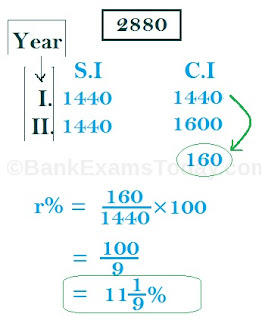New Students Offer - Use Code HELLO

# Simple Interest and Compound Interest Tricks

Today I am going to share Simple Interest and Compound Interest tricks and shortcuts.

## Simple Interest

### Formula of Simple Interest:### #1

Find the simple interest, If
1. P = Rs.1000, R = 20% per annum, T = 4 years.
2. P = Rs.600, R = 5% per annum, T = 4 months.
3. P = Rs.200, R = 6% per six months, T = 3 years.
4. P = Rs.500, R = 2% per six months, T = 5/2 years.
5. P = Rs.400, R = 3% per three months, T = 2 months.
6. P = Rs.730, R = 10% per annum, T = 120 days.
7. P = Rs. 3000, R = 61/4 per annum, T = period from 4th Feb to 18th Apr.

### # Solution

1. 4×20×10 ⇒ 800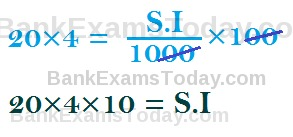2. 2×5 = 103. 6×2×3×2 = 724. 5×2×5=505. 4×2=86. 73/3=247. 37.50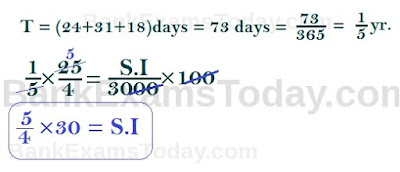### #2

Find the following:
1. P = Rs. 100, R = 3% per annum, T = 2 year, A= ?
2. P = Rs. 500, R = 6% per annum, T = 4 months, A= ?
3. P = Rs. 400, R = 3.65% per annum, T = 150 days, A= ?
4. A = Rs. 540, S.I = Rs. 108 , R = 5%, T = ?
5. A = Rs. 1,120, R = 5%, T = 22/5 yr, S.I = ?

### # Solution:

1. S.I = 6 ; A = S.I + principal ; A = 6 + 100 ⇒ 1062. S.I = 10 ; A = S.I + P ; A = 10+500 ⇒ 510
3. S.I = 6 ; A = 400 + 6 ⇒ 4064. T = 5 yr.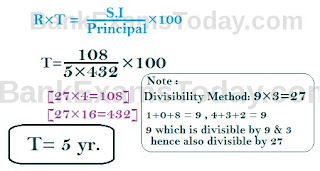5. 120### #3

1. A sum of money lent out at simple interest amounts to Rs. 720 after 2 years and to Rs. 1020 after a further period of 5 years. Find the sum and the rate %.
2. Adam borrowed some money at the rate of 6% p.a. for the first two years, at the rate of 9% p.a. for the next three years, and at the rate of 14% p.a. for the period beyond five years. If he pays a total interest of Rs. 11,400 at the end of nine years , how much money did he borrow ?(Bank P.O 1999)
3. A person borrows Rs. 5000 for 2 years at 4% p.a. simple interest. He immediately lends it to another person at 61/4% p.a. for 2 years. Find his gain in the transaction per year.(S.S.C.2000)
4. A certain sum of money amounts to Rs. 1008 in 2 years and to Rs. 1164 in 31/2 years.Find the sum and the rate of interest?
5. The simple interest on a certain sum of money for 21/2 years at 12% per annum is Rs. 40 less than the simple interest on the same sum for 31/2 years at 10% per annum. Find the sum.

### # Solution

1. Principal = 600, R = 10%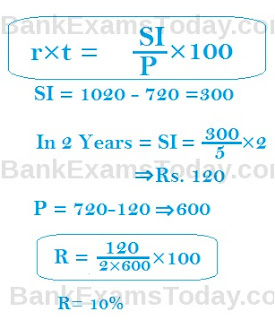2. 12000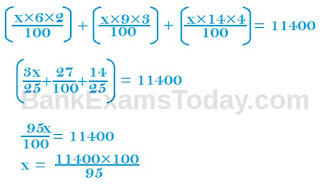3. 112.50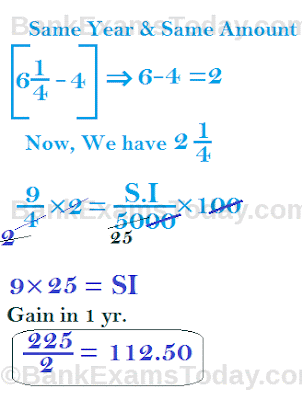4. [ 1164-1008 = 156  ] ⇒ 156/3×4 = 208 ; R = 208/2×800×100  13
5. 7x/20 - 3x/10 = 40 ⇒ x = ( 40 × 20 ) ⇒ x = 800 [ Hint : Given Below ]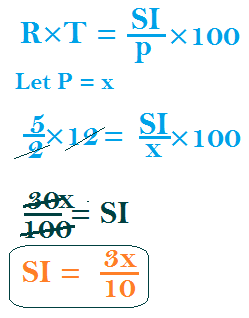## Compound Interest

### Formula of Compound Interest:### Trick :

#### Calculating Compound Interest for 3 Years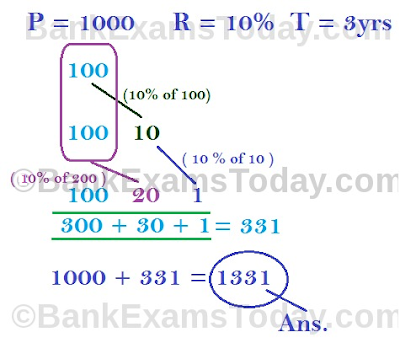#### Calculating Compound Interest for 4 year### # Formulas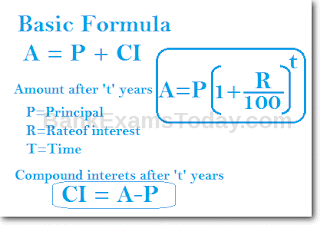Case 1. When interest is not Compound yearly,
Amount after 't' years A = P [1+ r/n×100]nt
n= no of compounding per year
When interest is compounded half yearly, n = 2
compounded quarterly, n = 4
compounded monthly, n = 12

Case 2. When rate % is no equal every year and interest is compounded yearly
Basic formula :
P [1+ r/100] [1+ r/100] ...upto 't' times
But as rate % is not same every year, so
A = P [1+ r1/100]t1 [1+ r2/100]t2 .... and so on
Where R1 = Rate% p.a. for t1 years. and R2 = Rate % p.a. for t2 years.
Case 3 When interest is compounded yearly but time is in fraction
T = 53/4 years
A= (whole part) × (fraction part of time )
A = P [1+ r/100]×  [1+ 3r/4/100]

### # Difference between Compound Interest and Simple Interest

CI - SI = P [ R/100 ]2
When time t = 3 years
CI - SI = P [ (R/100+3 (R/100)2]

### #1

• If the compound interest on a certain sum for two years at 10% p.a. is Rs 2,100 the simple interest on it at the same rate for two years will be. ( RRB, 2009)
• The compound interest on a sum for 2 years is Rs. 832 and the simple interest on the same sum for the same period is Rs. 800. The difference between the compound and simple interest for 3 years will be.
• The difference between simple interest and compound interest on a sum for 2 years at 8% when the interest is compounded annually is Rs. 16, if the interest were compounded half yearly, the difference in one interest would be nearly.
• The difference in C.I and S.I for 2 years on a sum of money is Rs. 160.If the S.I for 2 years be Rs. 2880, the rate of percent is .

### # Solution

1.  20002.  98.56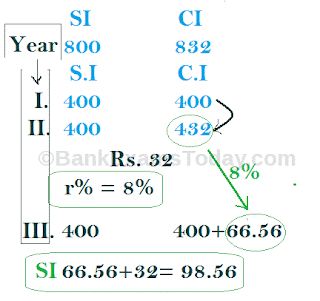3.  04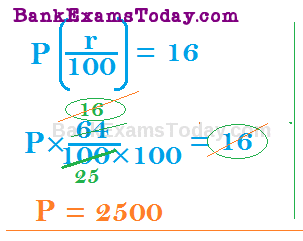4.